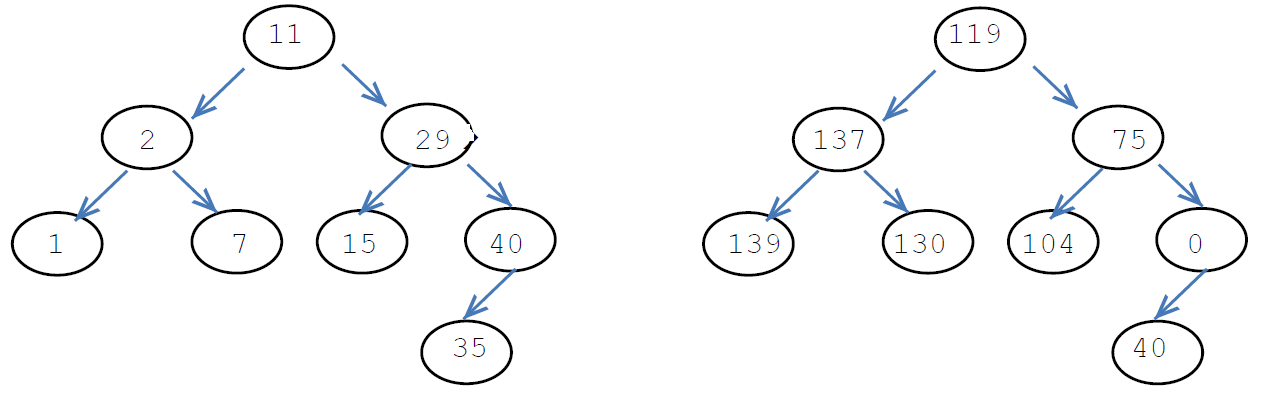4

# Convert Bst To The Greater Sum Tree

Difficulty: MEDIUMContributed By
Ankush Gupta
Avg. time to solve
30 min
Success Rate
70%

Problem Statement
Suggest Edit

#### Note :

``````You need to modify the given tree only. You are not allowed to create a new tree.
``````
##### For example:
``````For the given binary search tree
````````````11 will be replaced by {15 + 29 + 35 + 40}, i.e. 119.
2 will be replaced by {7 + 11 + 15 + 29 + 35 + 40}, i.e. 137.
29 will be replaced by {35 + 40}, i.e. 75.
1 will be replaced by {2 + 7 + 11 + 15 + 29 + 35 + 40}, i.e. 139.
7 will be replaced by {11 + 15 + 29 + 35 + 40}, i.e. 130.
15 will be replaced by {15 + 29 + 35 + 40}, i.e. 104.
40 will be replaced by 0 {as there is no node with a value greater than 40}.
35 will be replaced by {40}, i.e. 40.
``````
##### Input Format:
``````The first line contains an integer 'T', which denotes the number of test cases. Then the test cases follow.

The first line of each test case contains elements in the level order form. The line consists of values of nodes separated by a single space. In case a node is null, we take -1 on its place.

For example, the input for the tree depicted in the below image would be :
````````````1
2 3
4 -1 5 6
-1 7 -1 -1 -1 -1
-1 -1
``````

#### Explanation :

``````Level 1 :
The root node of the tree is 1

Level 2 :
Left child of 1 = 2
Right child of 1 = 3

Level 3 :
Left child of 2 = 4
Right child of 2 = null (-1)
Left child of 3 = 5
Right child of 3 = 6

Level 4 :
Left child of 4 = null (-1)
Right child of 4 = 7
Left child of 5 = null (-1)
Right child of 5 = null (-1)
Left child of 6 = null (-1)
Right child of 6 = null (-1)

Level 5 :
Left child of 7 = null (-1)
Right child of 7 = null (-1)

The first not-null node(of the previous level) is treated as the parent of the first two nodes of the current level. The second not-null node (of the previous level) is treated as the parent node for the next two nodes of the current level and so on.
The input ends when all nodes at the last level are null(-1).
``````
##### Note :
``````The above format was just to provide clarity on how the input is formed for a given tree.
The sequence will be put together in a single line separated by a single space. Hence, for the above-depicted tree, the input will be given as:

1 2 3 4 -1 5 6 -1 7 -1 -1 -1 -1 -1 -1
``````
##### Output Format:
``````For each test case, print a single line that contains the level order traversal of the greater sum tree where all nodes are printed in a single-space separated manner.

The output of each test case will be printed in a separate line.
``````
##### Note :
``````You do not need to print anything; it has already been taken care of. Just implement the given function.
``````
##### Constraints:
``````1 <= 'T' <= 100
0 <= 'N' <= 1000
0 <= 'DATA' <= 10 ^ 4 and 'DATA' != -1

Where ‘N’ is the total number of nodes in the binary search tree, and 'DATA' is the value of the binary search tree node.

Time Limit: 1sec
``````
##### Sample Input 1:
``````2
5 2 7 1 3 6 8 -1 -1 -1 -1 -1 -1 -1 -1
4 2 5 -1 3 -1 6 -1 -1 -1 -1
``````
##### Sample Output 1:
``````21 29 8 31 26 15 0
11 18 6 15 0
``````
##### Explanation of Sample Input 1:
``````For the first test case, after converting the given binary tree into the greater sum tree, the level order traversal of the tree will be {21, 29, 8, 31, 26, 15, 0}, where 5 will be replaced by {6+7+8} i.e. 21, 3 will be replaced by {5+6+7+8} i.e. 26, 7 will be replaced by {8} i.e. 8, 1 will be replaced by {2+3+5+6+7+8} i.e. 33, 2 will be replaced by {3+5+6+7+8} i.e. 26, 6 will be replaced by {7+8} i.e. 15 and 8 will be replaced by 0 (because no node has a value greater than 8 in the given tree).

For the second test case, after converting the given binary tree into the greater sum tree, the level order traversal of the tree will be {11, 18, 6, 15, 0}, where, 4 will be replaced by {5+6} i.e. 11, 2 will be replaced by {3+4+5+6} i.e. 18, 5 will be replaced by {6} i.e. 6, 3 will be replaced by {4+5+6} i.e. 15 and 6 will be replaced by 0 (because no node has a value greater than 6 in the given tree).
``````
##### Sample Input 2:
``````2
11 2 29 1 7 15 40 -1 -1 -1 -1 -1 -1 35 -1 -1 -1
10 5 15 2 7 12 20 -1 -1 -1 -1 -1 -1 -1 -1
``````
##### Sample Output 2:
``````119 137 75 139 130 104 0 40
47 64 20 69 57 35 0
``````
##### Explanation of Sample Input 2:
``````For the first test case, after converting the given binary tree into the greater sum tree, the level order traversal of the tree will be {119, 137, 75. 130, 104, 0, 40}.

For the second test case, after converting the given binary tree into the greater sum tree, the level order traversal of the tree will be {47, 64, 20, 69, 57, 35, 0}.
``````Console